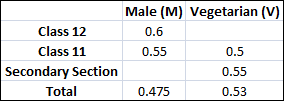# Tables with Missing Values for CAT PDF – Data Interpretation

0
5156

Tables with Missing Values questions, which are a type of Data Interpretation (DI) questions that often appear in the CAT exam. These questions require aspirants to analyze tables with missing data and use their mathematical and logical reasoning skills to fill in the missing values. The PDF includes detailed solutions and explanations to help CAT aspirants practice and improve their proficiency in solving Table with Missing Values-related problems.

Tables with Missing Values for CAT PDF:

]

Instructions:

Directions for the following four questions:
The proportion of male students and the proportion of vegetarian students in a school are given below. The school has a total of 800 students, 80% of whom are in the Secondary Section and rest equally divided between Class 11 and 12.Question 1:

What is the percentage of male students in the secondary section?
a) 40
b) 45
c) 50
d) 55
e) 60

Question 2:

In Class 12, twenty five per cent of the vegetarians are male. What is the difference between the number of female vegetarians and male non-vegetarians?
a) less than 8
b) 10
c) 12
d) 14
e) 16

Question 3:

What is the percentage of vegetarian students in Class 12?
a) 40
b) 45
c) 50
d) 55
e) 60

Question 4:

In the Secondary Section, 50% of the males are vegetarian. Which of the following statements is correct?
a) Except vegetarian males, all other groups have same number of students.
b) Except non-vegetarian males, all other groups have same number of students.
c) Except vegetarian females, all other groups have same number of students.
d) Except non-vegetarian females, all other groups have same number of students.
e) All of the above groups have the same number of students.

Answers and Solutions for Tables with Missing Values for CAT:

Solutions:

According to given condition No of male students in 11,12 and in total are 44 , 48 and 380.
So No of males in secondary are 380-44-48=288.
288 students => 45% of total secondary students.

There are 640 secondary section students, 80 11th class students and 80 12th class students.
If the fraction of vegetarians in 12th class is x, then
80x + 80*0.5 + 640*0.55 = 0.53*800
=> x + 0.5 + 4.4 = 5.3 => x = 0.4
=> There are 32 vegetarian students in class 12.
25% of class 12 i.e 8 are males.
So there are 24 vegetarian female students.
No. of non-veg males = 40 and veg females=24. Required answer 40 – 24 = 16.

There are 640 secondary section students, 80 11th class students and 80 12th class students.
If the fraction of vegetarians in 12th class is x, then
80x + 80*0.5 + 640*0.55 = 0.53*800
=> x + 0.5 + 4.4 = 5.3 => x = 0.4
=> 40% are vegetarians in class 12.